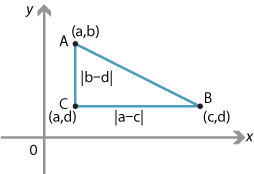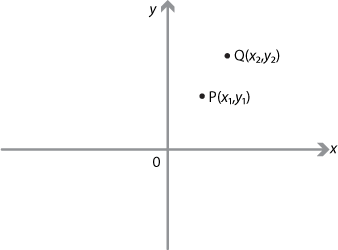## Content

### The distance between two points

Distances in geometry are always positive, except when the points coincide. The distance from $$A$$ to $$B$$ is the same as the distance from $$B$$ to $$A$$.

In order to derive the formula for the distance between two points in the plane, we consider two points $$A(a,b)$$ and $$B(c,d)$$. We can construct a right-angled triangle $$ABC$$, as shown in the following diagram, where the point $$C$$ has coordinates $$(a,d)$$.Detailed description

Now, using Pythagoras' theorem, we have

\begin{align*} AB^2 & = |b-d|^2 + |a-c|^2\\ &=(a-c)^2+(b-d)^2. \end{align*}

So

$AB = \sqrt{(a-c)^2+(b-d)^2}.$

A similar formula applies to three-dimensional space, as we shall discuss later in this module.

Interactive 1###### The distance formula

Suppose $$P(x_1,y_1)$$ and $$Q(x_2,y_2)$$ are two points in the number plane. Then

$PQ^2 = (x_2-x_1)^2+(y_2-y_1)^2$

and so

$PQ = \sqrt{(x_2-x_1)^2+(y_2-y_1)^2}.$It is clear from the distance formula that:

• $$PQ=QP$$
• $$PQ=0$$ if and only if $$P=Q$$.

Next page - Content - Midpoints and division of an interval